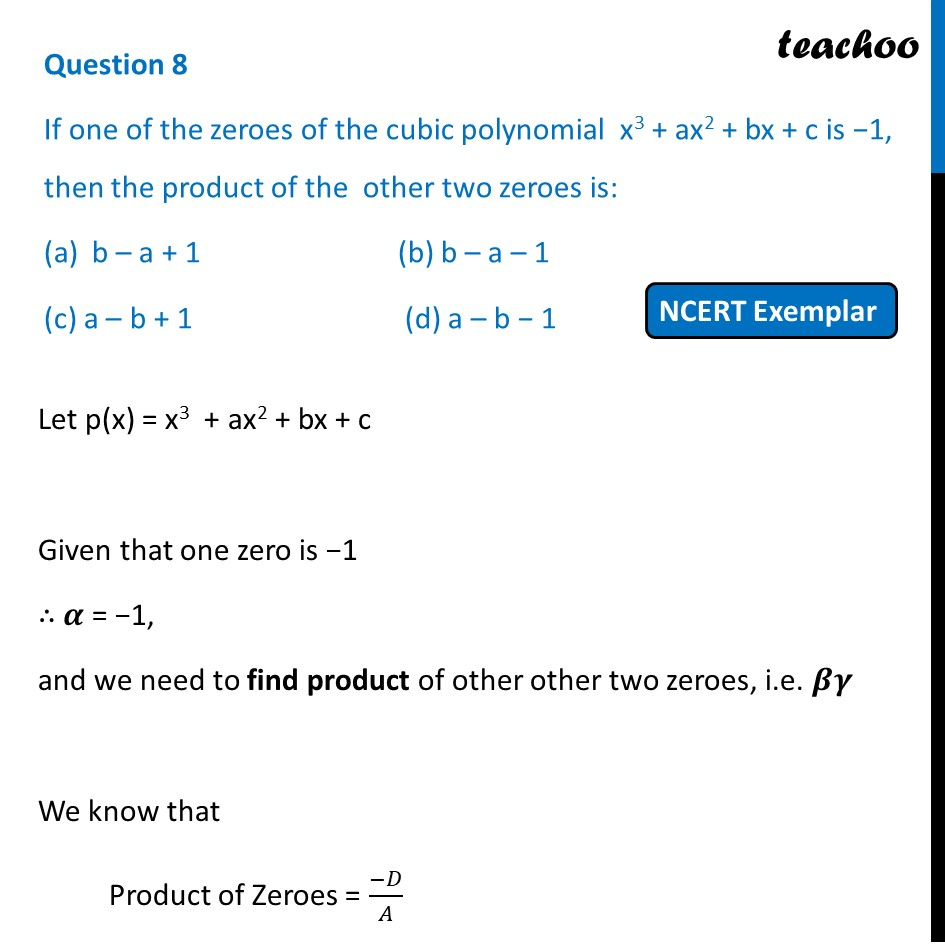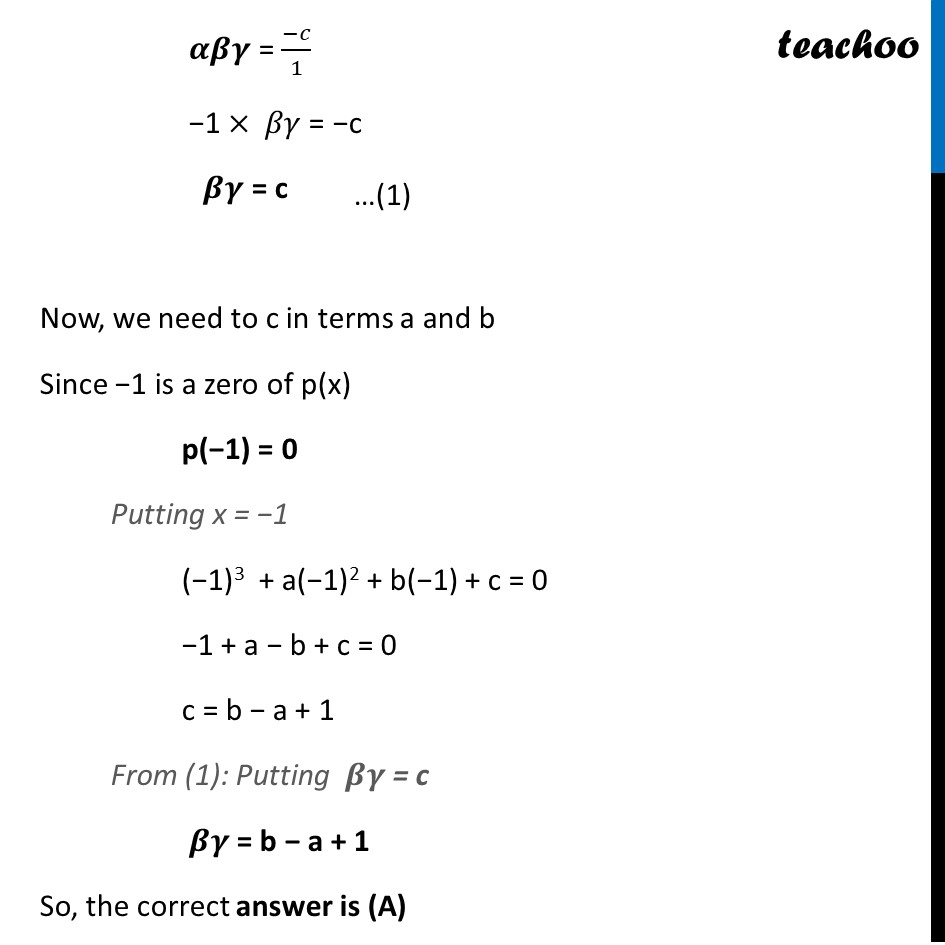## (c) a – b + 1  (d) a – b − 11. Chapter 2 Class 10 Polynomials (Term 1)
2. Serial order wise
3. MCQs from NCERT Exemplar

Transcript

Question 8 If one of the zeroes of the cubic polynomial x3 + ax2 + bx + c is −1, then the product of the other two zeroes is: b – a + 1 (b) b – a – 1 (c) a – b + 1 (d) a – b − 1 Let p(x) = x3 + ax2 + bx + c Given that one zero is −1 ∴ 𝜶 = −1, and we need to find product of other other two zeroes, i.e. 𝜷𝜸 We know that Product of Zeroes = (−𝐷)/𝐴 𝜶𝜷𝜸 = (−𝑐)/1 −1 × 𝛽𝛾 = −c 𝜷𝜸 = c Now, we need to c in terms a and b Since −1 is a zero of p(x) p(−1) = 0 Putting x = −1 (−1)3 + a(−1)2 + b(−1) + c = 0 −1 + a − b + c = 0 c = b − a + 1 From (1): Putting 𝜷𝜸 = c 𝜷𝜸 = b − a + 1 So, the correct answer is (A)

MCQs from NCERT Exemplar